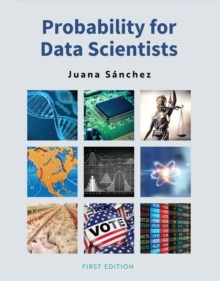Supporting your high street Find out how »
• My AccountProbability for Data Scientists Paperback / softback

Description

Probability for Data Scientists provides students with a mathematically sound yet accessible introduction to the theory and applications of probability.

Students learn how probability theory supports statistics, data science, and machine learning theory by enabling scientists to move beyond mere descriptions of data to inferences about specific populations. The book is divided into two parts. Part I introduces readers to fundamental definitions, theorems, and methods within the context of discrete sample spaces.

It addresses the origin of the mathematical study of probability, main concepts in modern probability theory, univariate and bivariate discrete probability models, and the multinomial distribution. Part II builds upon the knowledge imparted in Part I to present students with corresponding ideas in the context of continuous sample spaces.

It examines models for single and multiple continuous random variables and the application of probability theorems in statistics. Probability for Data Scientists effectively introduces students to key concepts in probability and demonstrates how a small set of methodologies can be applied to a plethora of contextually unrelated problems.

It is well suited for courses in statistics, data science, machine learning theory, or any course with an emphasis in probability.

Numerous exercises, some of which provide R software code to conduct experiments that illustrate the laws of probability, are provided in each chapter.

Information

• Format: Paperback / softback
• Pages: 362 pages
• Publisher: Cognella, Inc
• Publication Date:
• Category: Data analysis: general
• ISBN: 9781516532698

£88.95

£87.95

on all orders

Pick up orders

from local bookshops